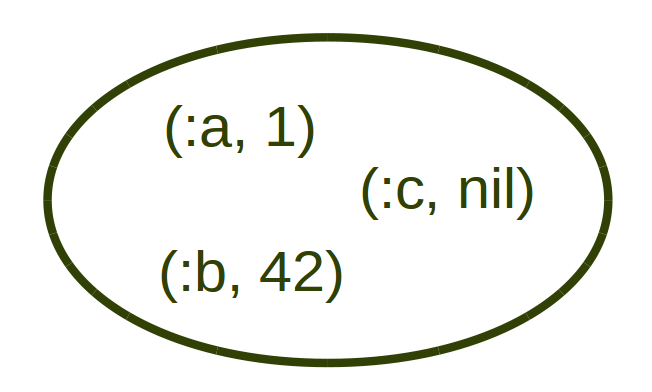Understanding Hash comparison in Ruby 2.3

by Paweł Świątkowski 20 Nov 2015

A first preview of Ruby 2.3 has been out for some time now. Of course, majority of attention is focused on incorporating Object.try! into language itself, but this is not the only new thing we have got with this release. I’ve read a few summaries about the new features and somehow they all missed a good explanation of the new Hash comparison operators. Every post I have seen gives examples of how it works but I haven’t seen one explaining why.

Now, after some analysis I think I know what it is about and here is my take on explaining that.

tl;dr

• a <= b – A is a subset of B
• a < b – A is a proper subset of B
• a == b – A and B are equal

Hashes as sets

As you can see above, I used terms from set theory to describe this behaviour. But how are the hashes sets? They can rather feel like some very discreet functions, assigning values to keys (arguments). But not necessarily. Let’s take a sample hash and represent it as a set of key-value pairs.As you see, it is quite simple. So, with this knowledge we can quite easily predict what the result of comparison will be. We can (and I will from now on) also represent this set in a bit more lisp-esque style: ((:a, 1) (:b, 42) (:c, nil)).

Subsets and proper subsets

By definition, set A is a subset of set B when every element of A is also an element of B. So let’s denote our example above as B and take on other set:

Is A a subset of B? Yes, because every (one) element of A is also an element of be. So A ⊆ B.

What about now? (:a, 1) is in both sets, but what about the second element? It doesn’t matter that first element is the same (stop thinking about it as a key – there is nothing special about it), (:b, 2) is not the same as (:b, 42) so A is not a subset of B. Let’s test our set intuition and understanding against Ruby 2.3:

The last two examples are also important. Remember that in set theory one of basic properties of empty set is that it is a subset of any set (including empty set as well). As we see above, this is also true in Ruby. Another maybe-a-bit-less-obvious thing is that every set is a subset of itself. And again: this is true.

The last statement may seem confusing. After all when you see sub you expect something less. It becomes clear when we take another look at the definition: every element of set A is also an element of set A, so it is it’s own subset. This is enough for most applications in set theory, but if you really care, you can define a proper subset term. As you probably already guessed, A is a proper subset of B when every element of A is an element of B, but A is not equal B.

This is denoted as A ⊊ B and in Ruby by a pair of operators: < and >. So:

Afterword

I know I didn’t cover equality, but this seems pretty obvious. I hope that explanation of hash comparison as set inclusion helps you better understand how this new feature of Ruby works, how to use it and how to reason about it.

Please, also bear in mind that even though you can express every Hash as a set of pairs, it does not work the other way round. For example ((:a, 1) (:a, 2)) is a totally valid set but also invalid Hash. But this is a completely different story and irrelevant for Hash comparison.

end of the article